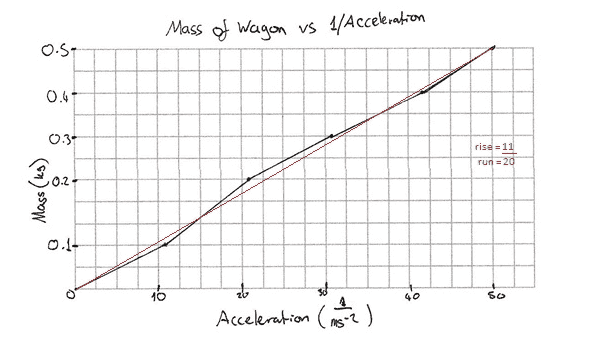# How does the gradient of the graph compare to the W force

## Homework Statement

How does the gradient of the graph compare to the weight force?
The graph is a Mass vs 1/Acceleration graph (y axis = mass, x axis = Acceleration, It was mentioned to do this.)

## Homework Equations

Explain by referring to the formula for Newton's Second Law.

## The Attempt at a Solution

I know that F = m * a
Could someone explain what must be done and what the use of a reciprocal is?

Thanks!

cnh1995
Homework Helper
Gold Member
How is the slope of a line represented in terms of x and y co-ordinates of a point on the line (assuming the line passes through (0,0))?

Hi,
The slope of the graph from the line of best fit is 11/20.
The problem I am having is with the comparison of the slope to the weight force which I calculated to be
W = m * g mass of 1 gram = 0.001 KG

W = 0.001 * 9.8

W = 0.0098 Nharuspex